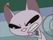cancel
Showing results for
Did you mean:## Quicker Way to do "MAXIFS" to Find the Last Purchase Date

Here's a sample of what I'm doing in EXCEL and need to translate into Power QueryThe first  3 columns are connected to an external data source (no formula required).

For every row (that represents an order) I want to know the corresponding previous order for that specific company.

I.E. US-158 (blue) is showing 4 orders, their first order (ID 3843) doesn't have a previous order so it's value is 0.  For their 2nd order (3848), the 4th column indicates that this company's previous order was 3843.  etc. etc.

In Excel, I set the formula in the [previous_order] column to find the maximum order_ID. Excel has the useful "MAXIFS" function so I can set the following conditions

1. Only look at the [order_ID] column when the value is less than the "order_ID" of that specific row.
2. Only look at rows where the [account_number] is the same as the "account_number" of that specific row.

The real data set has 20k purchase records.  In POWER QUERY, I've found a way to replicate it by grouping the rows according to account_number, adding a rank, adding a row of lists, then expanding each list.  The problem is that it takes 5-10 minutes to refresh in Power BI.  (I found 4 other ways, with grouping, conditional merging, "List.Max", etc.  - but they take even longer.)

Excel calculates all 20k records in 4 seconds.

Does anyone know a quicker way?

1 ACCEPTED SOLUTIONMemorable Member

Hi, @gogrizz try smth like this

``````let
Source = your_source_table,
g = Table.Group(Source, "account", {{"data", each f(Table.Sort(_, "order_id"))}}),
f = (t as table) as table =>
let
a = List.Buffer({0} & List.RemoveLastN(t[order_id], 1)),
b = Table.FromColumns(Table.ToColumns(t) & {a}, Table.ColumnNames(t) & {"previous_order"})
in b,
expand = Table.ExpandTableColumn(g, "data", {"order_id", "order_date", "previous_order"})
in
expand``````
4 REPLIES 4Memorable Member

Hi, @gogrizz try smth like this

``````let
Source = your_source_table,
g = Table.Group(Source, "account", {{"data", each f(Table.Sort(_, "order_id"))}}),
f = (t as table) as table =>
let
a = List.Buffer({0} & List.RemoveLastN(t[order_id], 1)),
b = Table.FromColumns(Table.ToColumns(t) & {a}, Table.ColumnNames(t) & {"previous_order"})
in b,
expand = Table.ExpandTableColumn(g, "data", {"order_id", "order_date", "previous_order"})
in
expand``````That's unreal.  Thank you so much!  I started down something similar, but I was no where near what you wrote.

I don't expect a full tutorial...but do you know of a video or site that goes through the general idea of what's going on there?  It works perfectly - but I can't figure out exactly why.

Thanks again!Memorable Member

Hi, the whole idea is to "slide down" order_id column by 1 position (skip last item and add zero item ahead). [order_id] column is a list so we use List.RemoveLastN function to cut the tail and lists concatenation ( & ) to add {0} element. To add the whole new column to our table we transform our table into a list of lists (of columns) using Table.ToColumns, add new list ( {a} ) and transform this list back into table using Table.FromColumns. This is how custom function f works.

In order to apply this function to a particular account data we group our data by account (Table.Group) and apply our function to each group of data (step g). Don't forget to sort every table by order_id (or order_date and order_id).

Finally we expand our (transformed) account data tables back into single table. One may use Table.Combine applied to the [data] column (this is list of tables) as well but I've chosen  Table.ExpandTableColumn this time.

It's all about the main idea (step a in custom function) and basic knowledge of how to work with tables and lists. It's a challenge every time. Have fun with Power Query M!Another huge help!  Thanks for going above and beyond!Announcements#### Exclusive opportunity for Women!

Join us for a free, hands-on Microsoft workshop led by women trainers for women where you will learn how to build a Dashboard in a Day!#### Power Platform Conference-Power BI and Fabric Sessions

Join us Oct 1 - 6 in Las Vegas for the Microsoft Power Platform Conference.Top Solution Authors
Top Kudoed Authors
Users online (3,321)